# Hyperbolic functions¶

## Hyperbolic functions¶

### cosh()¶

mpmath.cosh(x, **kwargs)

Computes the hyperbolic cosine of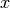,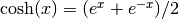. Values and limits include:

>>> from mpmath import *
>>> mp.dps = 25; mp.pretty = True
>>> cosh(0)
1.0
>>> cosh(1)
1.543080634815243778477906
>>> cosh(-inf), cosh(+inf)
(+inf, +inf)


The hyperbolic cosine is an even, convex function with a global minimum at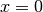, having a Maclaurin series that starts:

>>> nprint(chop(taylor(cosh, 0, 5)))
[1.0, 0.0, 0.5, 0.0, 0.0416667, 0.0]


Generalized to complex numbers, the hyperbolic cosine is equivalent to a cosine with the argument rotated in the imaginary direction, or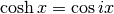:

>>> cosh(2+3j)
(-3.724545504915322565473971 + 0.5118225699873846088344638j)
>>> cos(3-2j)
(-3.724545504915322565473971 + 0.5118225699873846088344638j)


### sinh()¶

mpmath.sinh(x, **kwargs)

Computes the hyperbolic sine of,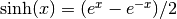. Values and limits include:

>>> from mpmath import *
>>> mp.dps = 25; mp.pretty = True
>>> sinh(0)
0.0
>>> sinh(1)
1.175201193643801456882382
>>> sinh(-inf), sinh(+inf)
(-inf, +inf)


The hyperbolic sine is an odd function, with a Maclaurin series that starts:

>>> nprint(chop(taylor(sinh, 0, 5)))
[0.0, 1.0, 0.0, 0.166667, 0.0, 0.00833333]


Generalized to complex numbers, the hyperbolic sine is essentially a sine with a rotationapplied to the argument; more precisely,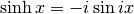:

>>> sinh(2+3j)
(-3.590564589985779952012565 + 0.5309210862485198052670401j)
>>> j*sin(3-2j)
(-3.590564589985779952012565 + 0.5309210862485198052670401j)


### tanh()¶

mpmath.tanh(x, **kwargs)

Computes the hyperbolic tangent of,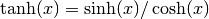. Values and limits include:

>>> from mpmath import *
>>> mp.dps = 25; mp.pretty = True
>>> tanh(0)
0.0
>>> tanh(1)
0.7615941559557648881194583
>>> tanh(-inf), tanh(inf)
(-1.0, 1.0)


The hyperbolic tangent is an odd, sigmoidal function, similar to the inverse tangent and error function. Its Maclaurin series is:

>>> nprint(chop(taylor(tanh, 0, 5)))
[0.0, 1.0, 0.0, -0.333333, 0.0, 0.133333]


Generalized to complex numbers, the hyperbolic tangent is essentially a tangent with a rotationapplied to the argument; more precisely,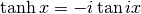:

>>> tanh(2+3j)
(0.9653858790221331242784803 - 0.009884375038322493720314034j)
>>> j*tan(3-2j)
(0.9653858790221331242784803 - 0.009884375038322493720314034j)


### sech()¶

mpmath.sech(x)

Computes the hyperbolic secant of,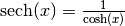.

### csch()¶

mpmath.csch(x)

Computes the hyperbolic cosecant of,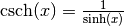.

### coth()¶

mpmath.coth(x)

Computes the hyperbolic cotangent of,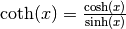.

## Inverse hyperbolic functions¶

### acosh()¶

mpmath.acosh(x, **kwargs)

Computes the inverse hyperbolic cosine of,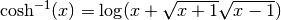.

### asinh()¶

mpmath.asinh(x, **kwargs)

Computes the inverse hyperbolic sine of,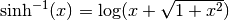.

### atanh()¶

mpmath.atanh(x, **kwargs)

Computes the inverse hyperbolic tangent of,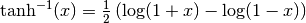.

### asech()¶

mpmath.asech(x)

Computes the inverse hyperbolic secant of,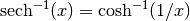.

### acsch()¶

mpmath.acsch(x)

Computes the inverse hyperbolic cosecant of,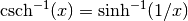.

### acoth()¶

mpmath.acoth(x)

Computes the inverse hyperbolic cotangent of,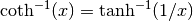.